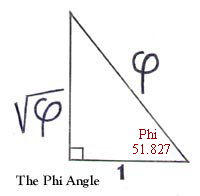PHI

Phi has a value equal to the

(square root of 5) + 1
2

The decimal equivalent = 1.61803 39887 49894 84821...
Phi may also be seen as the length of the hypotenuse in the right triangle having the other two sides equal to 1, and the square root of Phi.Using a2 + b2 = c2, Phi angle, I would suggest, could be seen as,
51 degrees 49'38.23"

From the trig tables...

 angle sin cos tan ctg sec csc 51 degrees 49' .61818 .78601 .78646 1.27153 1.27225 1.61765 51 degrees 50' .61795 .78622 .78598 1.27250 1.27191 1.61825 Phi .61803 .78615 .78615 1.27202 1.27202 1.61803 Phi -1 *** Phi -2 Phi -2 Phi 1/2 Phi 1/2 Phi

this Phi angle of 51 degrees 9'38.23"or 51.82729 degrees
is the only angle in trig chart where

cos = tan
ctg x sin = cos
cos2 = sin
cos x ctg = 1
ctg 2 = Phi

SOME PHI RELATED ANGLES
 ANGLE sin cos tan ctg tan+ctg ctg/tan 20 degrees 54' 18.585" * Phi -2 Phi 2 3 Phi 4 31 degrees 43' 02.908" ** cos(ctg) Phi(sin) Phi -1 *** Phi root 5 Phi 2 58 degrees 16' 57.092" Phi(cos) ctg(sin) Phi Phi -1 *** root 5 Phi -2 69 degrees 05' 41.415" Phi 2 Phi -2 3 Phi 4

* ½ angle from dodecahedron (10 zone diamond)
** ½ angle from icosahedron (6 zone diamond)
Diamonds are made by taking an edge and drawing radii from the end points. This gives you ½ of the diamond.
*** noting that Phi - 1 = 1/Phi = Phi-1

 Phi exponent decimal value -3 .23606 79775 -5/2 .30028 30967 -2 .38196 60113 -3/2 .48586 82568 -1 .61803 39887 -1/2 .78615 13535 1/2 1.27201 96888 1 1.61803 39887 3/2 2.05817 10908 2 2.61803 39887 5/2 3.33019 21120 3 4.23606 79775 4 6.85410 19662

Phi angles are seen in the basic structures of a pentagon and in the penrose tiles. Phi appears in all 5 fold polyhedra...
dodechedron, icosahedron, triacontahedron, enneacontahedron, truncated cuboctahedron, great rhombicuboctahedron, truncated icosadodecahedron, great rhombicosadodecahedron, pentagonal hexacontahedron among others.

BACK